# Normal space

(diff) ← Older revision | Latest revision (diff) | Newer revision → (diff)
No. Shorthand A topological space is said to be normal(-minus-Hausdorff) if ... A topological space$X$ is said to be normal(-minus-Hausdorff) if ...
1 separation of disjoint closed subsets by open subsets given any two disjoint closed subsets in the topological space, there are disjoint open sets containing them. given any two closed subsets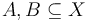$A,B \subseteq X$ such that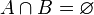$A \cap B = \varnothing$, there exist disjoint open subsets$U,V$ of$X$ such that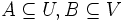$A \subseteq U, B \subseteq V$, and$U \cap V = \varnothing$.
2 separation of disjoint closed subsets by continuous functions given any two disjoint closed subsets, there is a continuous function taking the value$0$ at one closed set and 1 at the other. for any two closed subsets$A,B \subseteq X$, such that$A \cap B = \varnothing$, there exists a continuous map$f:X \to [0,1]$ (to the closed unit interval) such that$f(x) = 0 \ \forall x \in A$ and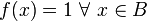$f(x) = 1 \ \forall \ x \in B$.
3 point-finite open cover has shrinking every point-finite open cover possesses a shrinking. for any point-finite open cover$U_i, i \in I$ of$X$, there exists a shrinking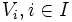$V_i, i \in I$: the$V_i$ form an open cover and$\overline{V_i} \subseteq U_i$.